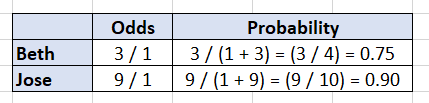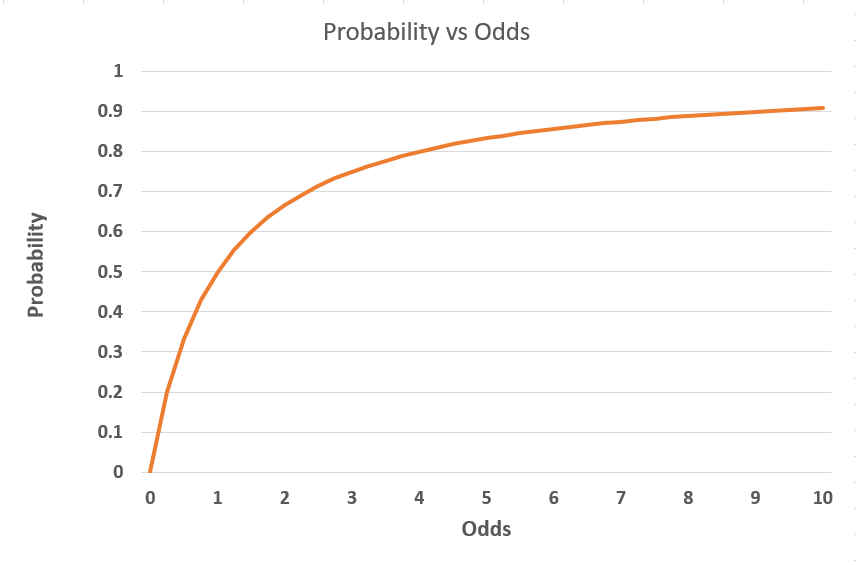What are the lifetime odds of dying from being hit by a meteorite?

1 in 1,600,000.

Yep, not very likely.  You are much more likely to die from a dog attack (1 in 86,781) or from a lightning strike (1 in 138,849).

But why odds?

Why not express these likelihoods in terms of probabilities?  Seems like a more natural way to express the chance of an event occurring, doesn’t it?

Odds, however, are commonly used to express event risk.  And of course, the chances of winning a sporting event.

As we write, the odds of the Los Angeles Dodgers repeating as World Series champions in 2021 are 1 in 3.

## So, what are odds?

The number of times an event occurs divided by the number of times it does not occur.

In the case of a meteorite strike, for every person that dies, 1.6 million do not.  In the case of the Dodgers, we would expect the Dodgers to win 1 World Series for every 3 they lose.

But this still begs the question, why odds and not probabilities?

## Odds and probabilities

It is true that the probability of a low likelihood event is so small that stating it as a % requires a lot of zeros after the decimal (0.0000625% in the case of dying from a meteorite strike).

But that is not an insurmountable objection. For example, the risk of disease is often expressed in terms of rates per 100,000 to make the chances of low likelihood events easier to comprehend. Or we could state the probability of the non-event…not dying from a meteorite strike (99.994%).

A more important reason for using odds is that they facilitate multiplicative comparisons.

A simple example makes clear how probabilities can fall short.

Suppose the probability that Beth will go out to dinner this weekend is 75%. We cannot say, then, that the probability of Jose doing the same is 3 times that of Beth’s probability.

Why?  Probabilities are constrained to lie between 0 and 1. And 3 * 0.75 > 1.0.

So, what do we do?  Enter odds.

### Odds are unconstrained

Odds are only bounded on the low end, by 0.  Let’s return to Beth and Jose.

The odds of Beth going out to dinner are 3 or 3/1.  Why 3/1?

Remember odds are the ratio of the events to non-events. Beth is 75% likely to go out. So, if she is faced with 4 opportunities to go out, she will do so 3 times.  In other words, she will go out (event) 3 times for every time she stays home (non-event).  3 to 1.

Now, if Jose is 3 times as likely to go out as Beth, his odds are simply 3 * 3 or 9.  Equivalently, we can express his odds as 9 to 1 or 9/1.

On the odds scale, odds can be 2, 10, 50 times greater…there is no upper limit. And this makes them very useful when we wish to compare the relative likelihood of events occurring.

### Two sides of the same coin

It turns out that if we are still interested in the probability, we can easily derive it from the odds.  Odds and probability are two sides of the same coin.

Odds (o) are related to probability (p) by the following:

o = p / (1 – p) = (probability of event / probability of non-event)

Rearranging we find the “other side of the coin” (for an event):

p = o / (1 + o) = (odds of event) / (1 + odds of event)

So, in the case of Beth and Jose we get:The relationship between odds and probability is shown graphically below.As the odds increase, the probability also increases but in a non-linear manner.  As shown above, the probability “increases at a decreasing rate” and approaches 1.0 “asymptotically” (i.e., as the odds get very large, the probability approaches but never quite reaches 1.0).

But any finite odds will map to a probability between 0 and 1.

## Odds are preferred

When comparing the relative chances of events (or sports teams), odds are the preferred way of expressing how much more likely one event is over another.  We can always derive the associated probability.  But since odds are unconstrained,  there is no issue with saying the Los Angeles Dodgers are 11 times as likely (as of May 31, 2021) to win the World Series in 2021 than the Chicago Cubs.

So, the next time someone tells you the odds of rain during your camping trip this weekend are 5 to 2, you might want to sleep in a tent.

 As of May 31, 2021, the reported odds of the Dodgers repeating are 3 to 1 or 3/1.  In the betting world, this is referred to as fractional odds. The number on the left or numerator is typically the number of times the team is expected to lose. 3/1 yields an implied probably of losing 3 times out of 4 or 75%.  Thus, the probability of the Dodgers repeating are (1 – 0.750) or 25%.  Expressing as odds yields 1/3.

 In the limit, if the odds = infinity, then probability = 1.

Topics: analytics#### Written by Kevin Duffy-Deno

Dr. Kevin Duffy-Deno, with over 30 years of experience in quantitative research and analysis, is an expert in econometric, data, statistical and predictive modeling and data visualization. Both with Bintel and prior organizations, Dr. Duffy-Deno has been engaged in numerous projects requiring data acquisition from proprietary and public sources, the normalization of such data and the integration of data into a format supporting analytic analyses and products.